Publicité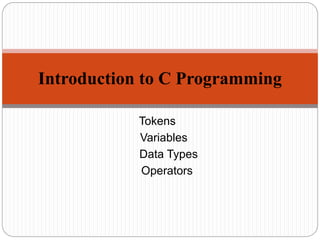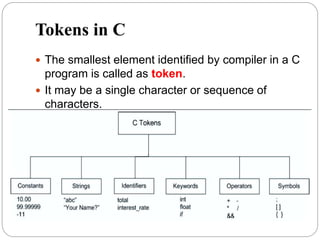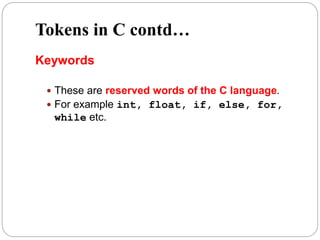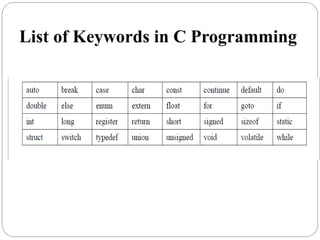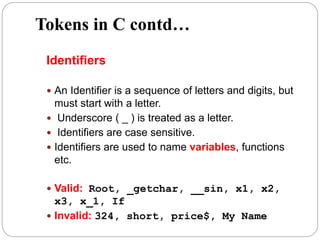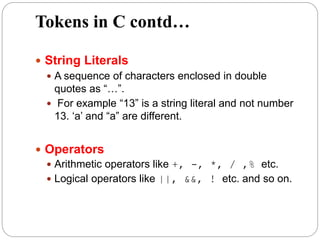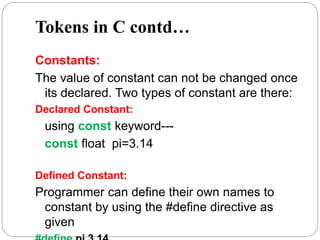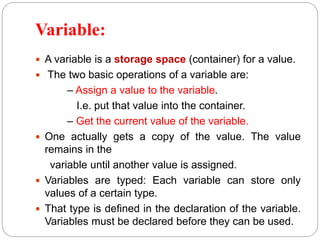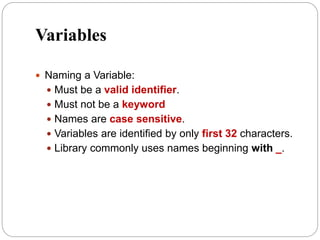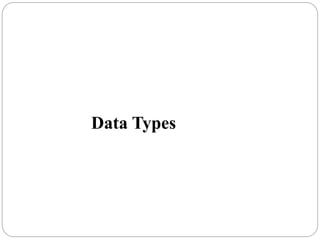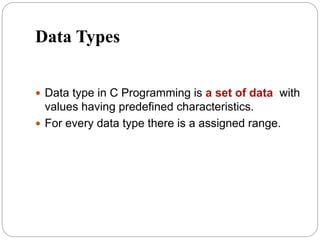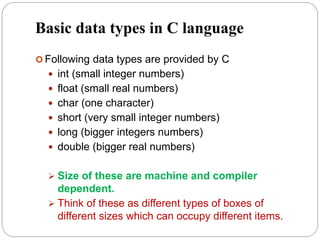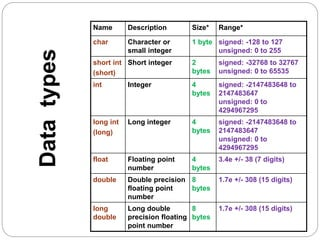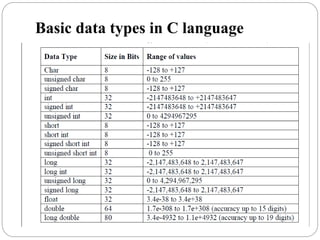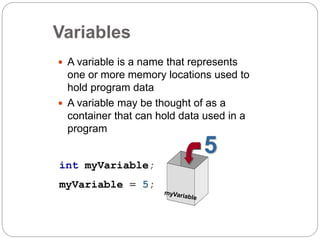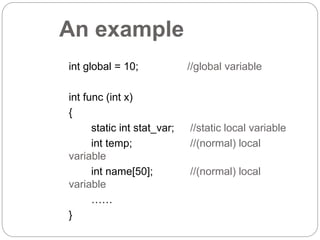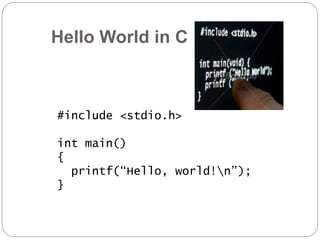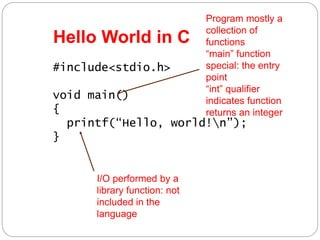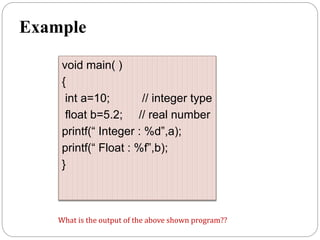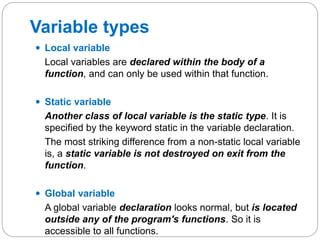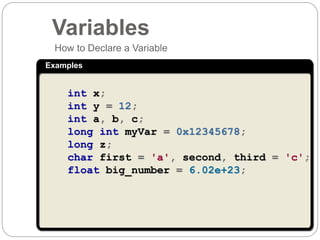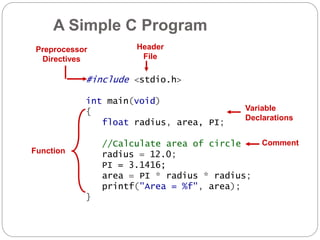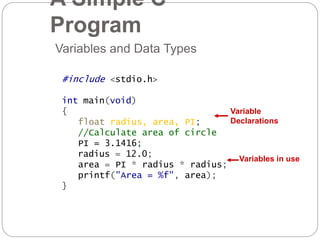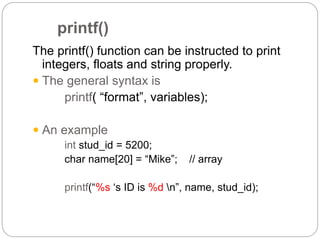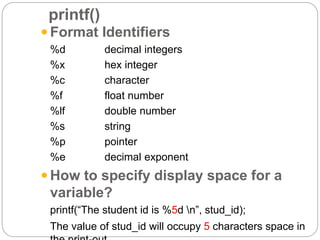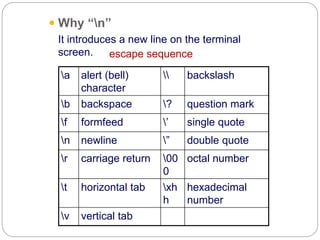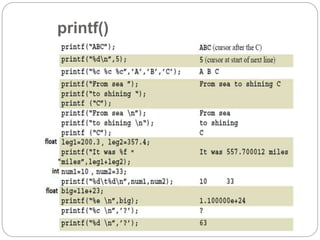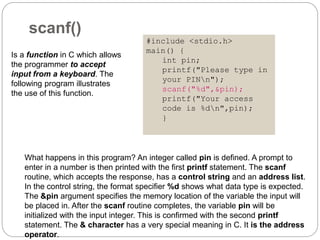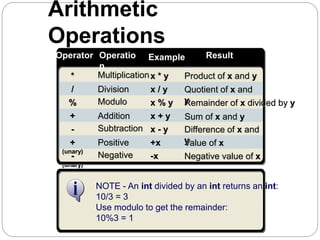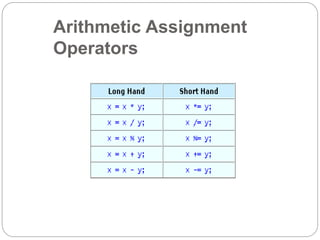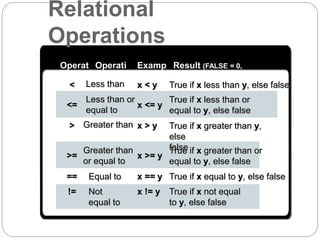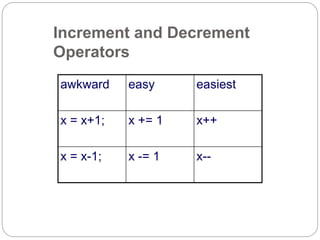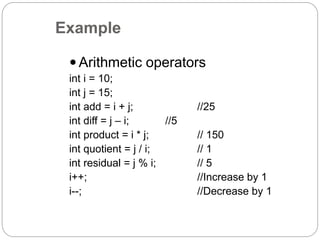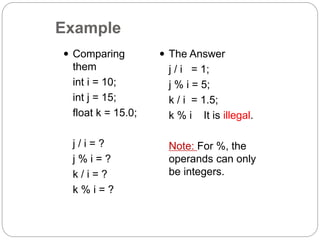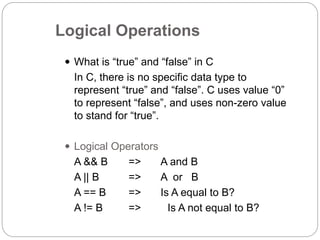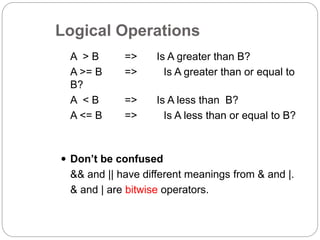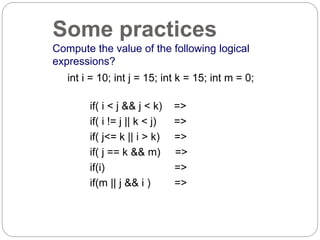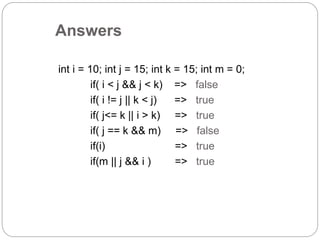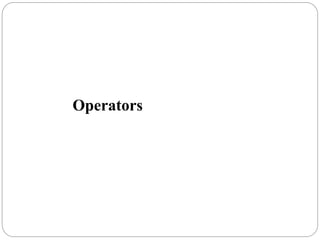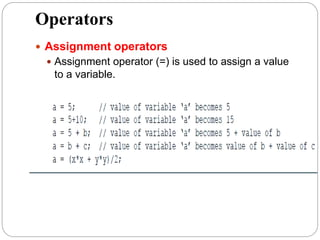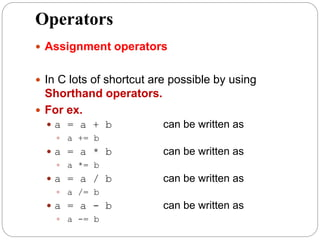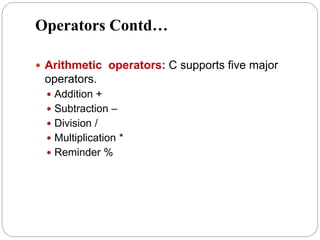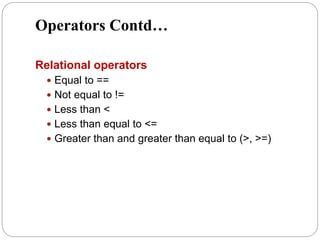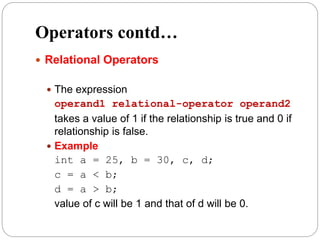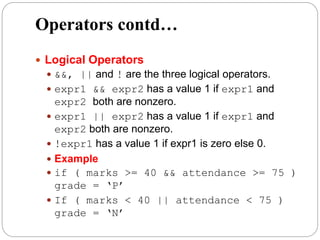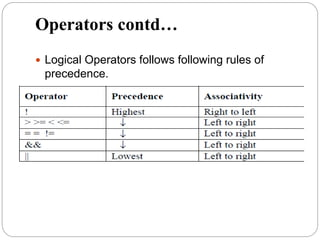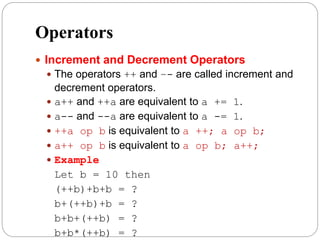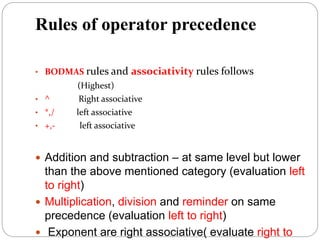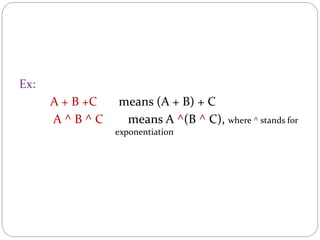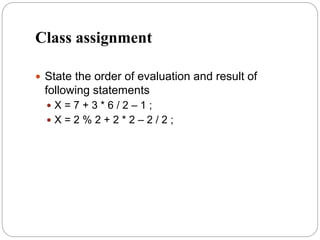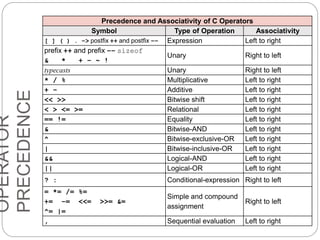1 sur 52
Publicité

### presentation_data_types_and_operators_1513499834_241350.pptx

1. Tokens Variables Data Types Operators Introduction to C Programming
2. Tokens in C  The smallest element identified by compiler in a C program is called as token.  It may be a single character or sequence of characters.
3. Tokens in C contd… Keywords  These are reserved words of the C language.  For example int, float, if, else, for, while etc.
4. List of Keywords in C Programming
5. Tokens in C contd… Identifiers  An Identifier is a sequence of letters and digits, but must start with a letter.  Underscore ( _ ) is treated as a letter.  Identifiers are case sensitive.  Identifiers are used to name variables, functions etc.  Valid: Root, _getchar, __sin, x1, x2, x3, x_1, If  Invalid: 324, short, price\$, My Name
6. Tokens in C contd…  String Literals  A sequence of characters enclosed in double quotes as “…”.  For example “13” is a string literal and not number 13. ‘a’ and “a” are different.  Operators  Arithmetic operators like +, -, *, / ,% etc.  Logical operators like ||, &&, ! etc. and so on.
7. Tokens in C contd… Constants: The value of constant can not be changed once its declared. Two types of constant are there: Declared Constant: using const keyword--- const float pi=3.14 Defined Constant: Programmer can define their own names to constant by using the #define directive as given
8. Variable:  A variable is a storage space (container) for a value.  The two basic operations of a variable are: – Assign a value to the variable. I.e. put that value into the container. – Get the current value of the variable.  One actually gets a copy of the value. The value remains in the variable until another value is assigned.  Variables are typed: Each variable can store only values of a certain type.  That type is defined in the declaration of the variable. Variables must be declared before they can be used.
9. Variables  Naming a Variable:  Must be a valid identifier.  Must not be a keyword  Names are case sensitive.  Variables are identified by only first 32 characters.  Library commonly uses names beginning with _.
10. Data Types
11. Data Types  Data type in C Programming is a set of data with values having predefined characteristics.  For every data type there is a assigned range.
12. Basic data types in C language  Following data types are provided by C  int (small integer numbers)  float (small real numbers)  char (one character)  short (very small integer numbers)  long (bigger integers numbers)  double (bigger real numbers)  Size of these are machine and compiler dependent.  Think of these as different types of boxes of different sizes which can occupy different items.
13. Name Description Size* Range* char Character or small integer 1 byte signed: -128 to 127 unsigned: 0 to 255 short int (short) Short integer 2 bytes signed: -32768 to 32767 unsigned: 0 to 65535 int Integer 4 bytes signed: -2147483648 to 2147483647 unsigned: 0 to 4294967295 long int (long) Long integer 4 bytes signed: -2147483648 to 2147483647 unsigned: 0 to 4294967295 float Floating point number 4 bytes 3.4e +/- 38 (7 digits) double Double precision floating point number 8 bytes 1.7e +/- 308 (15 digits) long double Long double precision floating point number 8 bytes 1.7e +/- 308 (15 digits) Data types
14. Basic data types in C language
15. Variables  A variable is a name that represents one or more memory locations used to hold program data  A variable may be thought of as a container that can hold data used in a program int myVariable; myVariable = 5; 5
16. An example int global = 10; //global variable int func (int x) { static int stat_var; //static local variable int temp; //(normal) local variable int name; //(normal) local variable …… }
17. Hello World in C #include <stdio.h> int main() { printf(“Hello, world!n”); }
18. Hello World in C #include<stdio.h> void main() { printf(“Hello, world!n”); } Program mostly a collection of functions “main” function special: the entry point “int” qualifier indicates function returns an integer I/O performed by a library function: not included in the language
19. Example void main( ) { int a=10; // integer type float b=5.2; // real number printf(“ Integer : %d”,a); printf(“ Float : %f”,b); } What is the output of the above shown program??
20.  Local variable Local variables are declared within the body of a function, and can only be used within that function.  Static variable Another class of local variable is the static type. It is specified by the keyword static in the variable declaration. The most striking difference from a non-static local variable is, a static variable is not destroyed on exit from the function.  Global variable A global variable declaration looks normal, but is located outside any of the program's functions. So it is accessible to all functions. Variable types
21. Examples How to Declare a Variable int x; int y = 12; int a, b, c; long int myVar = 0x12345678; long z; char first = 'a', second, third = 'c'; float big_number = 6.02e+23; Variables
22. A Simple C Program #include <stdio.h> int main(void) { float radius, area, PI; //Calculate area of circle radius = 12.0; PI = 3.1416; area = PI * radius * radius; printf("Area = %f", area); } Header File Function Variable Declarations Comment Preprocessor Directives
23. Variables and Data Types Variable Declarations Variables in use #include <stdio.h> int main(void) { float radius, area, PI; //Calculate area of circle PI = 3.1416; radius = 12.0; area = PI * radius * radius; printf("Area = %f", area); } A Simple C Program
24. printf() The printf() function can be instructed to print integers, floats and string properly.  The general syntax is printf( “format”, variables);  An example int stud_id = 5200; char name = “Mike”; // array printf(“%s ‘s ID is %d n”, name, stud_id);
25.  Format Identifiers %d decimal integers %x hex integer %c character %f float number %lf double number %s string %p pointer %e decimal exponent  How to specify display space for a variable? printf(“The student id is %5d n”, stud_id); The value of stud_id will occupy 5 characters space in printf()
26.  Why “n” It introduces a new line on the terminal screen. a alert (bell) character backslash b backspace ? question mark f formfeed ’ single quote n newline ” double quote r carriage return 00 0 octal number t horizontal tab xh h hexadecimal number v vertical tab escape sequence
27. printf()
28. Example: The following example shows the usage of scanf() function to read strings. Note: in scanf, we do not use & with array names #include <stdio.h> int main() { char str1, str2; printf("Enter name: "); scanf("%s", str1); printf("Enter your website name: "); scanf("%s", str2); printf("Entered Name: %sn", str1); printf("Entered Website:%s", str2); } scanf() OUTPUT : Enter name: raja Enter your website name: google.com Entered Name: raja Entered Website: google.com
29. What happens in this program? An integer called pin is defined. A prompt to enter in a number is then printed with the first printf statement. The scanf routine, which accepts the response, has a control string and an address list. In the control string, the format specifier %d shows what data type is expected. The &pin argument specifies the memory location of the variable the input will be placed in. After the scanf routine completes, the variable pin will be initialized with the input integer. This is confirmed with the second printf statement. The & character has a very special meaning in C. It is the address operator. Is a function in C which allows the programmer to accept input from a keyboard. The following program illustrates the use of this function. scanf() #include <stdio.h> main() { int pin; printf("Please type in your PINn"); scanf("%d",&pin); printf("Your access code is %dn",pin); }
30. Negative - (unary) Subtraction - Positive + (unary) Modulo % Addition + NOTE - An int divided by an int returns an int: 10/3 = 3 Use modulo to get the remainder: 10%3 = 1 Multiplication * Division / Operator Result Operatio n Example -x x - y +x x % y x + y x * y x / y Negative value of x Difference of x and y Value of x Remainder of x divided by y Sum of x and y Product of x and y Quotient of x and y Arithmetic Operations
31. Arithmetic Assignment Operators
32. Operat or Result (FALSE = 0, TRUE ≠ 0) Operati on Examp le Equal to == Not equal to != Greater than > Greater than or equal to >= Less than < Less than or equal to <= x == y x != y x > y x >= y x < y x <= y True if x equal to y, else false True if x not equal to y, else false True if x greater than y, else false True if x greater than or equal to y, else false True if x less than y, else false True if x less than or equal to y, else false Relational Operations
33. Increment and Decrement Operators awkward easy easiest x = x+1; x += 1 x++ x = x-1; x -= 1 x--
34. Example  Arithmetic operators int i = 10; int j = 15; int add = i + j; //25 int diff = j – i; //5 int product = i * j; // 150 int quotient = j / i; // 1 int residual = j % i; // 5 i++; //Increase by 1 i--; //Decrease by 1
35.  Comparing them int i = 10; int j = 15; float k = 15.0; j / i = ? j % i = ? k / i = ? k % i = ?  The Answer j / i = 1; j % i = 5; k / i = 1.5; k % i It is illegal. Note: For %, the operands can only be integers. Example
36. Logical Operations  What is “true” and “false” in C In C, there is no specific data type to represent “true” and “false”. C uses value “0” to represent “false”, and uses non-zero value to stand for “true”.  Logical Operators A && B => A and B A || B => A or B A == B => Is A equal to B? A != B => Is A not equal to B?
37. A > B => Is A greater than B? A >= B => Is A greater than or equal to B? A < B => Is A less than B? A <= B => Is A less than or equal to B?  Don’t be confused && and || have different meanings from & and |. & and | are bitwise operators. Logical Operations
38. Some practices Compute the value of the following logical expressions? int i = 10; int j = 15; int k = 15; int m = 0; if( i < j && j < k) => if( i != j || k < j) => if( j<= k || i > k) => if( j == k && m) => if(i) => if(m || j && i ) =>
39. int i = 10; int j = 15; int k = 15; int m = 0; if( i < j && j < k) => false if( i != j || k < j) => true if( j<= k || i > k) => true if( j == k && m) => false if(i) => true if(m || j && i ) => true Answers
40. Operators
41. Operators  Assignment operators  Assignment operator (=) is used to assign a value to a variable.
42. Operators  Assignment operators  In C lots of shortcut are possible by using Shorthand operators.  For ex.  a = a + b can be written as  a += b  a = a * b can be written as  a *= b  a = a / b can be written as  a /= b  a = a - b can be written as  a -= b
43. Operators Contd…  Arithmetic operators: C supports five major operators.  Addition +  Subtraction –  Division /  Multiplication *  Reminder %
44. Operators Contd… Relational operators  Equal to ==  Not equal to !=  Less than <  Less than equal to <=  Greater than and greater than equal to (>, >=)
45. Operators contd…  Relational Operators  The expression operand1 relational-operator operand2 takes a value of 1 if the relationship is true and 0 if relationship is false.  Example int a = 25, b = 30, c, d; c = a < b; d = a > b; value of c will be 1 and that of d will be 0.
46. Operators contd…  Logical Operators  &&, || and ! are the three logical operators.  expr1 && expr2 has a value 1 if expr1 and expr2 both are nonzero.  expr1 || expr2 has a value 1 if expr1 and expr2 both are nonzero.  !expr1 has a value 1 if expr1 is zero else 0.  Example  if ( marks >= 40 && attendance >= 75 ) grade = ‘P’  If ( marks < 40 || attendance < 75 ) grade = ‘N’
47. Operators contd…  Logical Operators follows following rules of precedence.
48. Operators  Increment and Decrement Operators  The operators ++ and –- are called increment and decrement operators.  a++ and ++a are equivalent to a += 1.  a-- and --a are equivalent to a -= 1.  ++a op b is equivalent to a ++; a op b;  a++ op b is equivalent to a op b; a++;  Example Let b = 10 then (++b)+b+b = ? b+(++b)+b = ? b+b+(++b) = ? b+b*(++b) = ?
49. Rules of operator precedence • BODMAS rules and associativity rules follows (Highest) • ^ Right associative • *,/ left associative • +,- left associative  Addition and subtraction – at same level but lower than the above mentioned category (evaluation left to right)  Multiplication, division and reminder on same precedence (evaluation left to right)  Exponent are right associative( evaluate right to
50. Ex: A + B +C means (A + B) + C A ^ B ^ C means A ^(B ^ C), where ^ stands for exponentiation
51. Class assignment  State the order of evaluation and result of following statements  X = 7 + 3 * 6 / 2 – 1 ;  X = 2 % 2 + 2 * 2 – 2 / 2 ;
52. Precedence and Associativity of C Operators Symbol Type of Operation Associativity [ ] ( ) . –> postfix ++ and postfix –– Expression Left to right prefix ++ and prefix –– sizeof & * + – ~ ! Unary Right to left typecasts Unary Right to left * / % Multiplicative Left to right + – Additive Left to right << >> Bitwise shift Left to right < > <= >= Relational Left to right == != Equality Left to right & Bitwise-AND Left to right ^ Bitwise-exclusive-OR Left to right | Bitwise-inclusive-OR Left to right && Logical-AND Left to right || Logical-OR Left to right ? : Conditional-expression Right to left = *= /= %= += –= <<= >>= &= ^= |= Simple and compound assignment Right to left , Sequential evaluation Left to right OPERATOR PRECEDENCE

1. 15
2. 21
3. 22
4. 23
5. 30
6. 32
Publicité